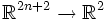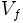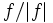Milnor map

Let$f$ be a complex polynomial in$(n+1)$ variables.$f$ can be viewed as a map$\R^{2n+2} \to \R^2$. Let$V_f$ denote the zero set of$f$. Then, on the complement of$V_f$, we can define a map$f/|f|$ from$\R^{2n+2} \setminus V_f$ to$S^1$.
The Milnor map of$f$ at radius$r$ is the restriction of this map to the sphere of radius$r$, centered at the origin, to$S^1$.
By the Milnor fibration theorem, the Milnor map is a fibration whenever the origin is an isolated singular point of$V_f$. Under such circumstances, it is termed the Milnor fibration.